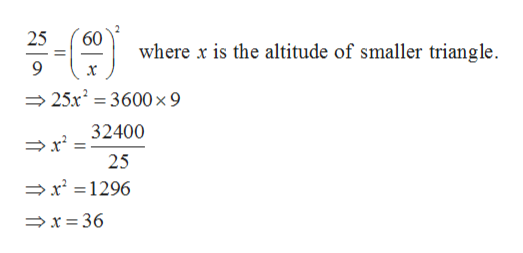# The areas of two similar triangles are in the ratio of 25:9. If the altitude of the larger triangle is 60, what is the length of the corresponding altitude of the smaller triangle?

Question
2 views

The areas of two similar triangles are in the ratio of 25:9. If the altitude of the larger triangle is 60, what is the length of the corresponding altitude of the smaller triangle?

check_circle

Step 1

Given, the areas of two similar triangles are in the ratio 25 : 9 and the altitude of the larger triangle is 60.

Step 2

We know that the ratio of the area of the two similar triangles is equal t...help_outlineImage Transcriptionclose60 25 where x is the altitude of smaller triangle. x 25x23600 x 9 32400 25 x 1296 >x = 36 fullscreen

### Want to see the full answer?

See Solution

#### Want to see this answer and more?

Solutions are written by subject experts who are available 24/7. Questions are typically answered within 1 hour.*

See Solution
*Response times may vary by subject and question.
Tagged in

### Triangles